# Synthesis and Characterisation of Gold-Silver nanoparticles

Abstract
Solutions of chloroauric acid and silver nitrate were reduced using sodium citrate to form nanoparticles of varying composition, whose optical properties and physical dimensions were measured, giving the result that the molar extinction coefficients of the solutions increased exponentially with decreasing mole fraction of gold in solution.

Introduction

The interest in nanoparticles has grown exponentially over recent years, as scientists strive to explore the variance in electronic, optical and chemical properties with particle size. In this experiment, gold nanoparticles were made. There has been a lot of interest in gold nanoparticles over the years, ever since they were first produced by Sir Michael Faraday in 1857[i].

Gold nanoparticles can be produced by reduction of chloroauric acid (H[AuCl4]). After dissolving H[AuCl4], the solution heated while a reducing agent is added. This causes Au3+ ions to be reduced to neutral gold atoms. As more and more of these gold atoms form, the solution becomes supersaturated, and gold gradually starts to precipitate in the form of sub-nanometer particles. The rest of the gold atoms that form stick to the existing particles, and, if the solution is stirred vigorously enough, the particles will be fairly uniform in size.

The simplest way to make gold nanoparticles is to reduce a gold containing complex with citrate to produce brightly coloured nanoparticles solutions. These colours originate from the formation of a plasmon resonance band. A plasmon is a collective excitation of the electronic “fluid” in a piece of conducting material, like ripples on the surface of a pond are a collective mode of the water molecules of the liquid. Like water, the electronic fluid in a metal is close to incompressible. If the surface of a pond is pushed down with a float, the density of the water doesn’t change; instead water elsewhere is displaced, because the water molecules have finite volume and push each other out of the way. The electronic fluid acts similarly, not because of any finite size or even the Coulomb repulsion of the electrons, but mostly because of the Pauli Exclusion Principle, which tends to keep the electrons out of each others’ way.

These electronic ripples can have a well-defined wavelength (which is related to their momentum via the De Broglie equation). When the electrons are displaced, the positive charge left behind exerts an attractive force on the electrons, trying to pull them back to their original positions. This interaction is what makes the plasmons oscillate once they’re excited, giving them a frequency, and these Coulomb interactions are also why plasmons cost energy to excite. These Coulomb interactions with the positive background charge also force plasmons to obey certain boundary conditions at the edges of the host metal. As a result, nanoparticles can have discrete allowed plasmonic modes strongly influenced by particle shape, while larger structures (e.g., thin metal films) can have propagating plasmon modes over a broad range of wavelengths. Typical plasmon frequencies are comparable to the frequencies of visible light (i.e., ~ 1015 Hz). Plasmons decay (into incoherent electron-hole pair excitations), eventually dissipating their energy as the electrons scatter instead of oscillating smoothly, and as oscillating electric dipoles (and other multipoles) radiate.

Silver-gold bimetallic nanoparticles were also studied to investigate the effect of variations in the amounts of the two metals in each nanoparticle had on the optical properties of the solutions.

The aims of this experiment were to learn how the changing composition of nanoparticles can affect their optical properties, and to show how the properties of nanoparticles vary from those of ordinary chemical compounds by comparison of their optical properties with a metal complex.

Experimental

Preparation of gold nanoparticles

Gold solution (HAuCl4 (aq)) (1.00% w/w, 0.025 M, 1 mL) was added to a 10 mL volumetric flask and made up to the mark with distilled water. From this solution, now 0.0025 M, 2.5 mL was added to a conical flask and distilled water (90 mL) was added. From here on in, this solution will be known as solution 0. The solution was brought to the boil, and then aqueous sodium citrate was added (1% w/w, 5 mL). The mixture was allowed to boil for thirty minutes, during which time the colour changed from gold to a deep red. The solution was then cooled to room temperature, transferred to a 100 mL volumetric flask and made up to the mark with distilled water.

Preparation of gold-silver bimetallic nanoparticles and silver nanoparticles

Using the remainder of the diluted 0.0025 M gold solution and a 0.0025 M silver nitrate solution, the amounts shown in table 1 were added to conical flasks. Distilled water (90 mL) was added to each flask before bringing them to the boil and adding aqueous sodium citrate (1% w/w, 5 mL) to each boiling solution. The solutions were left to boil for thirty minutes, during which time the solutions turned red. The more gold that was in solution, the more red the solution was. The mixtures were then cooled to room temperature, then made up to 100 mL with distilled water in a volumetric flask.

The nanoparticles were characterised using UV-vis spectroscopy between 300 – 700 nm using water as a reference solution. A Malvern Zetasizer was used to measure the average size of particles in solution using dynamic light scattering.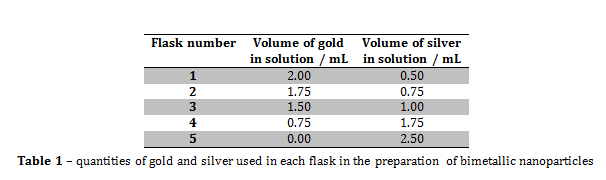Application of gold nanoparticles as sensors

Pure gold nanoparticle solution (5 mL) was placed into each of two large test tubes. To one of them, aqueous sodium chloride solution (1 M) was added dropwise. The solution went from deep red to grey when at least ten drops of NaCl had been added. The process was repeated with the second nanoparticle solution using saturated sodium chloride. The colour change in this test tube took only two drops of NaCl.

Results and Discussion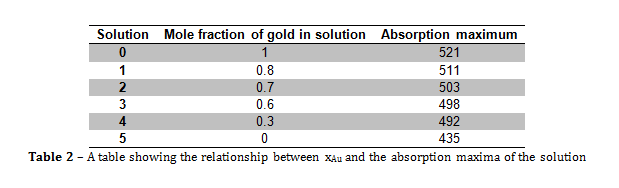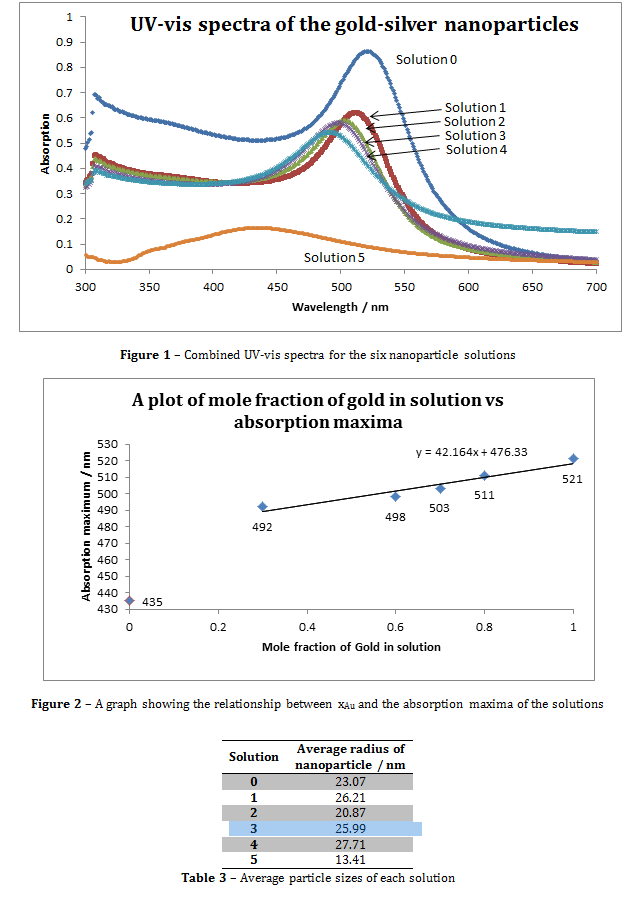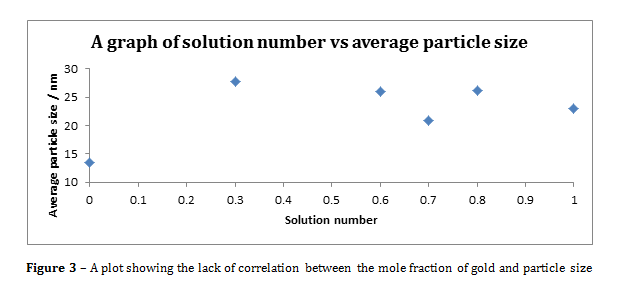Figure 3 – A plot showing the lack of correlation between the mole fraction of gold and particle size

From the information portrayed in figures 1 and 2, and in table 2, the absorption maximum of the solutions decreased with decreasing mole fraction of gold (xAu­) in solution, and there was only one absorption maximum for each solution. The fact that there was only one absorption maximum for each solution suggests that, even though there were two metals in solutions 1 to 4, they formed some kind of composite nanoparticle.

From figure 2, the line of best fit predicts that if xAu = 0 and xAg = 1, then the absorption maximum would be 476.33 nm, however, in this experiment, when xAu = 0 and xAg = 1, λmax = 435 nm, and the literature value was 375 nm. The value predicted by the line of best fit is vastly higher than the literature value, which suggests that the presence of gold within the nanoparticles greatly increases the value of λmax.

To calculate the molar extinction coefficients for the solutions, the average number of gold particles per nanoparticle must first be calculated using equation 1. The molar concentration is then determined using equation 2. Then the extinction coefficient be calculated using the Beer-Lambert law (equation 3)[i].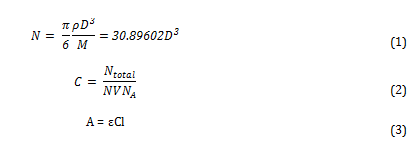Where N is the average number of gold atoms per nanoparticle, ρ is the density of fcc gold (g cm-3), D is the average diameter of the nanoparticle, displayed in table 3 and calculated using the Malvern zetasizer, M is the atomic weight of gold (197 g mol-1), C is the molar concentration, Ntotal is the initial amount of gold added to the solution, V is the volume of the reaction, NA is Avogadro’s number, A is λmax, ε is the molar extinction coefficient and l is the path length, which was 1cm in all cases.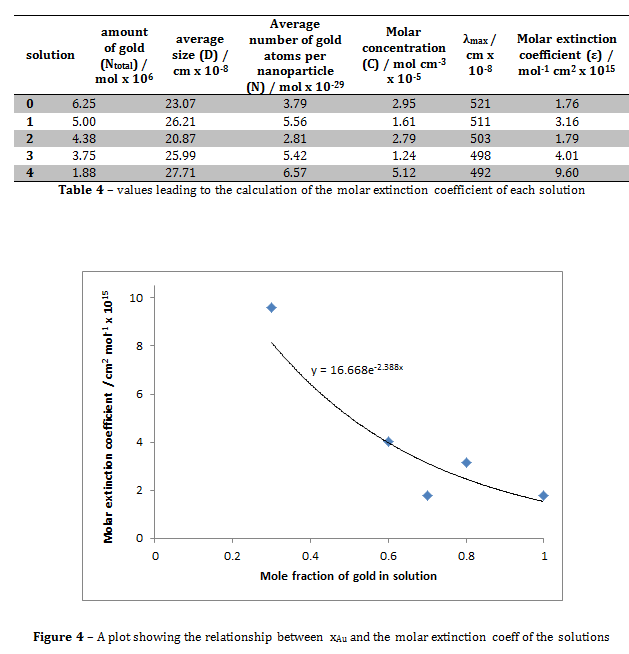Figure 4 shows that the relationship between the mole fraction of gold in solution and the molar extinction coefficient of the solution is roughly exponential. The molar extinction coefficient for the solution where xAu = 1 in this experiment was 1.76 x 10-15, compared to the literature value of (3.61 ± 0.08) x 106 ii. These values are clearly hugely different. This may be due to the slight differences in experimental technique. For example, in the journal, the reducing agent was decanethiol, whereas in this experiment the reducing agent used was sodium citrate. This may have altered the optical properties of the solution, hence giving the solution a different molar extinction coefficient.

There were no observations of larger gold particles forming. The reason for this is that when the nanoparticles form, the citrate anions were adsorbed onto their surface. This repels the gold particles from each other, preventing aggregation.

The absorption at λmax­ for the pure silver solution was not strong. It is difficult to make silver nanoparticles by citrate reduction because the products formed are often mixtures of spherical and rod shaped nanoparticles due to the poor balance of nucleation and growth processes[i]. Other methods of forming silver sols by other methods are possible though, for example, by the γ-irradiation of deaerated solutions containing 50 or 100 pM AgCl04[ii].

The zetasizer measures average particle size by using dynamic light scattering. The machine measured the diffusion of particles moving under Brownian motion and converts this to a size distribution using the Stokes-Einstein relationship[iii].

When sodium chloride was added to the solutions of gold colloids, the colour changed from a deep red to grey. Increasing the concentration of sodium chloride makes the colour change much quicker. This means that the reaction that occurs is directly related to the concentration of sodium chloride. The reaction that occurs is the destabilisation of the gold nanoparticles, where the sodium cations react with the citrate anions, exposing the surface of the gold nanoparticles, and changing their optical properties.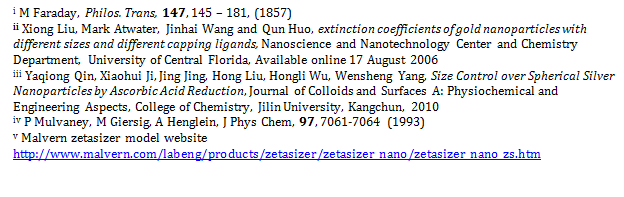• Share